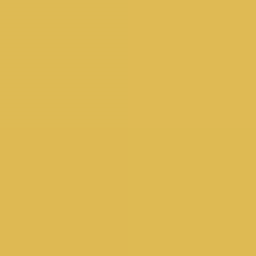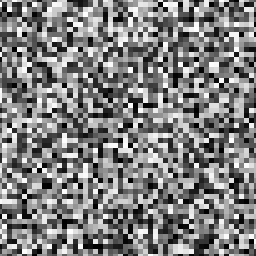Beta Feature. To enable, check Enable Beta Features in Preferences.

The Material Sampler does not currently support SkSL Shaders.

SkSL ("Skia Shading Language") is a variant of GLSL which is used as Skia's internal shading language. With some minor modifications, GLSL code from sites like https://www.shadertoy.com/ can be converted for use in Cavalry.

For more detail on the differences between GLSL and SkSL see the Skia documentation.

Note - our SkSL shader currently only takes float uniforms. We will be expanding this functionality soon.

## UI

Blend Mode - see Blend Modes.

Shader Code - paste/edit SkSL code here.

Inputs - click the `+ Add` button to to add a uniform.

## Examples

Have a play with a selection of example .cv files. 👇

Original source code:

Example usage:

1. Create a Rectangle

2. In the Attribute Editor, open the Fill tab.

4. Double click the SkSL Shader to load its Attribute Editor UI.

5. Copy and paste the example code below into the Shader Code attribute.

6. Add 3 inputs and ensure each has a value ≠ 0.

You should see a blue flame appear on the Rectangle. You can now start to play with connecting Behaviours (Noise, Sound etc) into each of the inputs.😎

`uniform float iTime;uniform float2 iResolution;​float noise(float3 p) //Thx to Las^Mercury{	float3 i = floor(p);	float4 a = dot(i, float3(1., 57., 21.)) + float4(0., 57., 21., 78.);	float3 f = cos((p-i)*acos(-1.))*(-.5)+.5;	a = mix(sin(cos(a)*a),sin(cos(1.+a)*(1.+a)), f.x);	a.xy = mix(a.xz, a.yw, f.y);	return mix(a.x, a.y, f.z);}​float sphere(float3 p, float4 spr){	return length(spr.xyz-p) - spr.w;}​float flame(float3 p){	float d = sphere(p*float3(1.,.3,1.), float4(.0,-1.,.0,1.));	return d + (noise(p+float3(.0,iTime*2.,.0)) + noise(p*3.)*.5)*.25*(p.y) ;}​float scene(float3 p){	return min(100.-length(p) , abs(flame(p)) );}​float4 raymarch(float3 org, float3 dir){	float d = 0.0, glow = 0.0, eps = 0.02;	float3  p = org;	bool glowed = false;		for(int i=0; i<64; i++)	{		d = scene(p) + eps;		p += d * dir;		if( d>eps )		{			if(flame(p) < .0)				glowed=true;			if(glowed)       			glow = float(i)/64.;		}	}	return float4(p,glow);}​half4 main(float2 fragCoord){	float2 v = -1.0 + 2.0 * fragCoord.xy / iResolution.xy;	v.x *= iResolution.x/iResolution.y;		float3 org = float3(0., -4.5, 4.);	float3 dir = normalize(float3(v.x*1.6, -v.y, -1.5));		float4 p = raymarch(org, dir);	float glow = p.w;		float4 col = mix(float4(1.,.5,.1,1.), float4(0.1,.5,1.,1.), p.y*.02+.4);		return half4(mix(float4(0.), col, pow(glow*2.,4.)));}`

Flat colour

Uniforms required = 0

`half4 main(float2 fragCoord) { return half4(0.385,0.775,1.000,1.000);}`

### Cycling colour

Uniforms required = 1

`0: Time``uniform float u_time;float3 colorA = float3(0.149,0.141,0.912);float3 colorB = float3(1.000,0.833,0.224);​half4 main(float2 fragCoord) { float3 color = float3(0.0);float percent = abs(sin(u_time*.01));// Mix uses percent (a value from 0–1) to // mix the two colors color = mix(colorA, colorB, percent);  return half4(color,1.0);}`

Pixels:Uniforms required = 3.

```0: Resolution.x 1: Resolution.y 2: Time```

`uniform float2 u_resolution;uniform float u_time;​float random (float2 st) { return fract(sin(dot(st.xy , float2(12.9898,78.233))) * 43758.5453);}​half4 main(float2 fragCoord) { float2 st = fragCoord / u_resolution.xy; st *= max(1.0, u_time); // Scale the coordinate system by 10 float2 ipos = floor(st); // get the integer coords float2 fpos = fract(st); // get the fractional coords// Assign a random value based on the integer coord float3 color = float3(random( ipos )); // Uncomment to see the subdivided grid // color = vec3(fpos,0.0);  return half4(color,1.0);}`

### Fractal Brownian motionUniforms required = 3.

```0: Resolution.x 1: Resolution.y 2: Time```

`uniform float2 u_resolution;uniform float u_time;​float random (in float2 _st) {    return fract(sin(dot(_st.xy, float2(12.9898,78.233))) * 43758.5453123);}​// Based on Morgan McGuire @morgan3d// https://www.shadertoy.com/view/4dS3Wdfloat noise (in float2 _st) {    float2 i = floor(_st);    // Four corners in 2D of a tile    float2 f = fract(_st);    float a = random(i);    float b = random(i + float2(1.0, 0.0));    float c = random(i + float2(0.0, 1.0));    float d = random(i + float2(1.0, 1.0));    float2 u = (f * f * (3.0 - 2.0 * f));    return mix(a, b, u.x) + (c - a) * u.y * (1.0 - u.x) +        (d - b) * u.x * u.y;}​float fbm ( in float2 _st) {    float v = 0.0;    float a = 0.5;    float2 shift = float2(100.0);    // Rotate to reduce axial bias    float2x2 rot = float2x2(cos(0.5), sin(0.5), -sin(0.5), cos(0.50));    for (int i = 0; i < 5; ++i) {        v += a * noise(_st);        _st = rot * _st * 2.0 + shift;        a *= 0.5;    }    return v;}​half4 main(float2 fragCoord) {    float2 st = fragCoord.xy/u_resolution.xy*3.;    // st += st * abs(sin(u_time*0.1)*3.0);    float3 color = float3(0.0);float2 q = float2(0.);    q.x = fbm( st + 0.00*u_time);    q.y = fbm( st + float2(1.0));float2 r = float2(0.);    r.x = fbm( st + 1.0*q + float2(1.7,9.2)+ 0.15*u_time );    r.y = fbm( st + 1.0*q + float2(8.3,2.8)+ 0.126*u_time);    float f = fbm(st+r);        color = mix(float3(0.101961,0.619608,0.666667), float3(0.666667,0.666667,0.498039),        clamp((f*f)*4.0,0.0,1.0));        color = mix(color,    float3(0,0,0.164706), clamp(length(q),0.0,1.0));    color = mix(color,float3(0.666667,1,1),clamp(length(r.x),0.0,1.0));    return half4((f*f*f+.6*f*f+.5*f)*color,1.);}`

Contents
UI
Examples